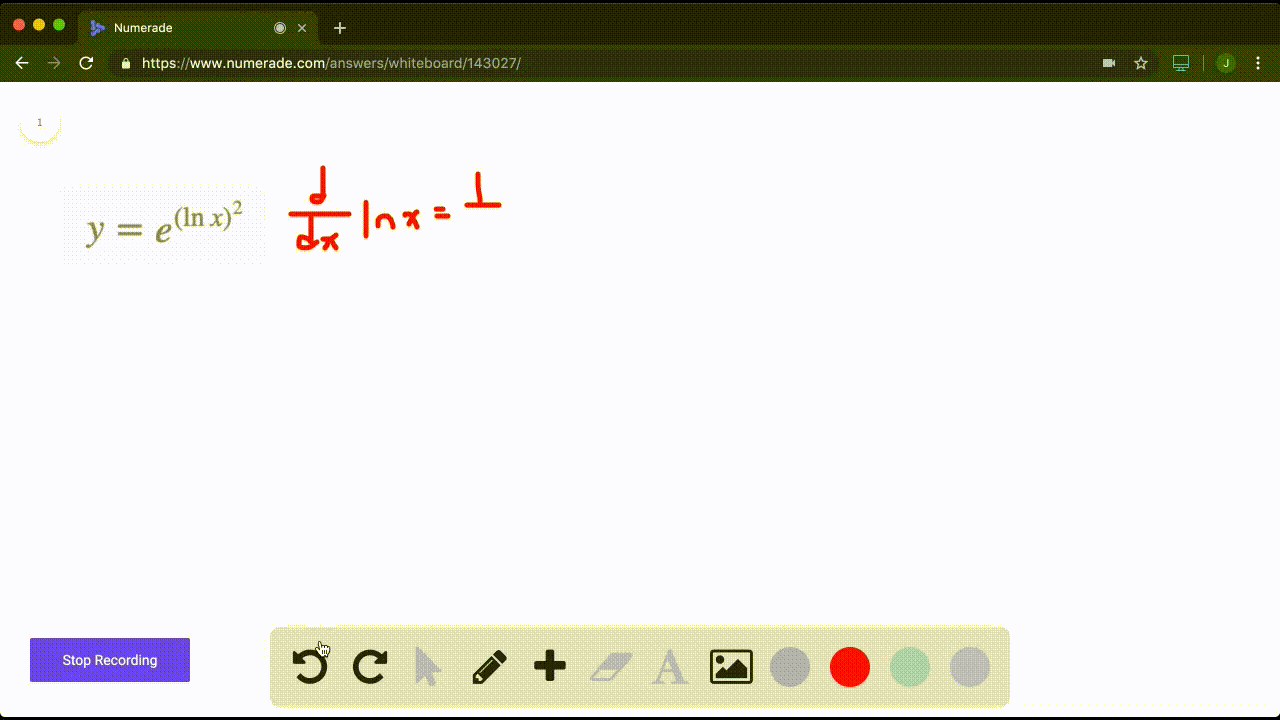Enroll in one of our FREE online STEM summer camps. Space is limited so join now!View Summer Courses### In Exercises $1-20,$ find the derivative. $$y=… ViewOther Schools Need more help? Fill out this quick form to get professional live tutoring. Get live tutoring Problem 9 In Exercises 1-20, find the derivative.$$
y=\frac{\ln x}{1}


$y^{\prime}=\frac{1-\ln x}{x^{2}}$

## Discussion

You must be signed in to discuss.

## Video Transcript

All right, so we're continuing to work on the exercises from 3.9, So this one's looking at question nine itself. But before you can do those, you need to be very comfortable with thes rules for differentiation. You would have been using them up until now. So just going through them briefly, You've got your directive of Constance. What happens if I have addition, subtraction, multiplication and division as well as what happens if I have functions of functions and how to deal with powers where I've got a variable base. We also added in this chapter are derivatives for exponential on log rhythmic functions. So what does the derivative of the e function look like versus a power with a variable exponents versus natural logs and regular logs? So, looking at this problem here, I've got a natural log I have to deal with as well as divisions. I'm gonna have to use my division rules or my quotient rules. So remember, with division I've got my two functions my top function, my bottom function, my numerator, my denominator. I'm gonna take the drivel of the first, multiply it by the regular second, subtract the derivative of my bottom multiplied by my regular top and then divide that all by my regular bottom squared. So I'm gonna have to deal with that division rule on how that plays in. And I'm also gonna have to deal with my natural log. So going through that the derivative, my natural log is just one over X. So those are the two that are gonna take place in this kind of equation, and we'll work through accordingly. So if I look at it my F function or my first function, my top one is my natural log. So I take the derivative of that which is one over X on Multiply that by my regular function on the bottom, which is just X. Then I subtract the derivative. My bottom, the derivative of X becomes technically, we move the one down and then it becomes one minus one, leaving it to a power of zero. But what happens with that X is I'm just left behind with the one I'd multiply that by my regular top, my f of X without the derivative. And then I have to divide this whole thing by my bottom functions squared. Now, cleaning this up a bit because we can we notice Here, I've got one over x times X So one times x x divided by ex that cancels thes out. So I'd be left with one there and then I don't have to write the one out front of the natural log. I can just leave. That is a natural log. And then I'd have my X squared still in the bottom. That I can't do anything with and not here would be how I would solve this. There's not much more I can do with that. That's vote is nice and clean as I can get it. And that's kind of how I apply my division rules with my natural log rules.